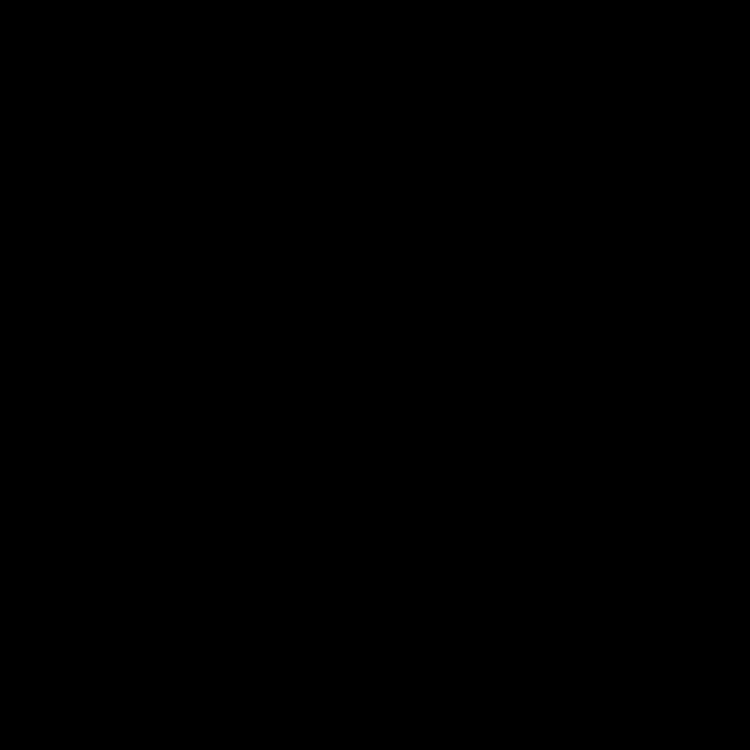# 65537 gon

Updated on
Covid-19
 Type  Regular polygonSchläfli symbol  {65537}Dual polygon  Self Edges and vertices  65537Internal angle (degrees)  ≈179.99°Symmetry group  Dihedral (D65537), order 2×65537

In geometry, a 65537-gon is a polygon with 65537 sides. The sum of the interior angles of any non-self-intersecting 65537-gon is 11796300°.

## Regular 65537-gon

The area of a regular 65537-gon is (with t = edge length)

A = 65537 4 t 2 cot π 65537

A whole regular 65537-gon is not visually discernible from a circle, and its perimeter differs from that of the circumscribed circle by about 15 parts per billion.

## Construction

The regular 65537-gon (one with all sides equal and all angles equal) is of interest for being a constructible polygon: that is, it can be constructed using a compass and an unmarked straightedge. This is because 65537 is a Fermat prime, being of the form 22n + 1 (in this case n = 4). Thus, the values cos π 65537 and cos 2 π 65537 are of a 32768-degree algebraic numbers, and like any constructible numbers they can be written in terms of square roots and no higher-order roots.

Although it was known to Gauss by 1801 that the regular 65537-gon was constructible, the first explicit construction of a regular 65537-gon was given by Johann Gustav Hermes (1894). The construction is very complex; Hermes spent 10 years completing the 200-page manuscript. Another method involves the use of at most 1332 Carlyle circles, and the first stages of this method are pictured below. This method faces practical problems, as one of these Carlyle circles solves the quadratic equation x2 + x − 16384 = 0 (16384 being 214).

## Symmetry

The regular 65537-gon has Dih65537 symmetry, order 131074. Since 65537 is a prime number there is one subgroup with dihedral symmetry: Dih1, and 2 cyclic group symmetries: Z65537, and Z1.

## 65537-gram

A 65537-gram is a 65537-sided star polygon. As 65537 is prime, there are 32767 regular forms generated by Schläfli symbols {65537/n} for all integers 2 ≤ n ≤ 32768 as 65537 2 = 32768 .

65537-gon Wikipedia

Topics